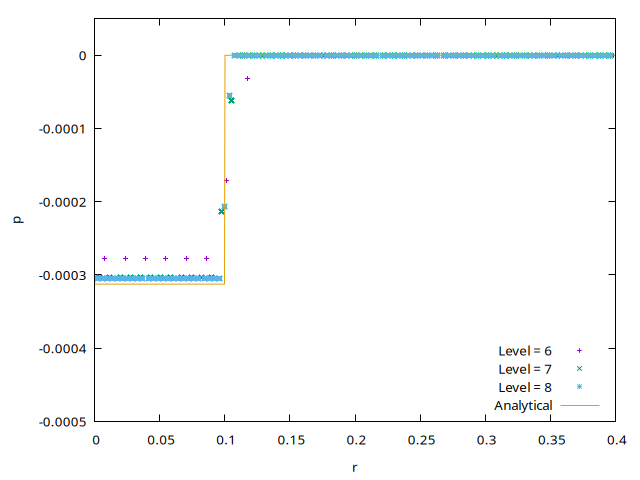# Charge relaxation in an axisymmetric insulated conducting column

A conducting rigid cylinder of radius R_o=0.1 is immersed in an insulating medium. Initially an uniform charge volume density is set in the cylinder (\rho_e (\mathbf{x},0)=0.5). As time passes the charge migrates from the bulk to the interface of the cylinder but the total charge in the cylinder section is preserved. \displaystyle Q(t)= \int_{\Sigma} \rho_e(\mathbf{x},t) \, d \Sigma = Q_o= \pi R_o^2 \, \rho_e(\mathbf{x},0). The outer electric field reaches a steady-state.

A more detailed discussion of this simulation is given in Lopez-Herrera et al, 2011.

The charges, initially uniformly distributed, tend to accumulate at the interface due to electrostatic repulsion (charge relaxation).

Since the total charge at the column remains constant, the electric potential distribution at the outer medium will also remain constant.

If the macro NAVIER is set to 1 a true EHD problem is solved otherwise the problem is purely electrostatic. The ohmic conduction is calculated implicitly although the conservative explicit scheme could also be used.

#define NAVIER 1
int LEVEL;

#include "grid/multigrid.h"
#include "axi.h"
#if NAVIER
# include "navier-stokes/centered.h"
# include "ehd/implicit.h"
# include "ehd/stress.h"
#else
# include "ehd/implicit.h"
#endif
#include "fractions.h"

Far away from the conducting column the electric potential is zero.

phi[top]   = dirichlet(0);
#if NAVIER
p[top]     = dirichlet(0);
u.n[top]   = neumann(0);
#endif

#define beta 3.
#define cond  3.
#define rhoini 0.5
#define R 0.1
#define A (R*R*rhoini/2.)
#define cylinder(y) (R - y)

scalar f[];

event init (t = 0) {

The conducting media will be defined through the scalar f. This will be useful also to define the electrical properties of the media and the initial distribution of charge density.

  face vector s[];
vertex scalar psi[];
foreach_vertex ()
psi[] = cylinder(y);
boundary ({psi});
fractions (psi, f, s);
foreach()
rhoe[] = rhoini*f[];
boundary ({rhoe});

The electrical conductivity and permittivity are defined on faces. The face permittivity is constructed from the center values of the VOF scalar f by averaging. On the other hand, face conductivity is set using the reconstructed surface fractions s.

Note that the electrostatic properties \varepsilon and K, i.e electrical permittivity and conductivity, are multiplied by the face metric factors.

  foreach_face(){
double ff = (f[] + f[-1])/2.;
epsilon.x[] = (ff*beta + (1. - ff))*fm.x[];
K.x[] = cond*s.x[]*fm.x[];
}
boundary ((scalar *){epsilon, K});
}

This event checks the conservation of the total charge.

event chargesum (i++) {
double Q = statsf(rhoe).sum;
static double Q0;
if (i == 0)
Q0 = Q;
else
assert (fabs(Q - Q0) < 1e-7);
}

At the final instant, when the charge is fully relaxed on the interface, the radial electric field and the pressure distribution are output in order to compare with analytical results.

event epfield (t = 10) {

The name of the file with the results is formed with the concatenation of Er with the corresponding level.

  char name;
sprintf (name, "Er-%d", LEVEL);
FILE * fp = fopen (name, "w");
scalar ee[];
foreach() {
double Ey = (phi[0,-1] - phi[0,1])/(2*Delta);
ee[] = Ey - (y < R ? 0. : 0.5*sq(R)*rhoini/y);
#if NAVIER
fprintf (fp, "%g %g %g %g\n", y, Ey, p[], rhoe[]);
#else
fprintf (fp, "%g %g %g\n", y, Ey, rhoe[]);
#endif
}
fclose (fp);

We also log the error on the norm of the electric field.

  norm n = normf (ee);
fprintf (stderr, "%d %g %g %g\n", LEVEL, n.avg, n.rms, n.max);
}

int main() {
X0 = -0.5;
L0 = 1.;
DT = 1;
TOLERANCE = 1e-7;
for (LEVEL = 6; LEVEL <= 8; LEVEL++) {
N = 1 << LEVEL;
run();
}
}
set term PNG enhanced font ",10"
set output 're.png'
set xlabel 'r'
set ylabel 'E_r'
set xrange[0:1]
set yrange[0.:0.03]
set sample 1000
R = 0.1
rhoini = 0.5
E(x) = x < R ? 0 : (R*R*rhoini/2/x)
plot 'Er-6' u 1:2 t "Level = 6",  \
'Er-7' u 1:2 t "Level = 7",  \
'Er-8' u 1:2 t "Level = 8",  \
E(x) w l t "Analytical"Radial electric field distribution as a function of grid refinement. (script)

set output 'p.png'
set xlabel 'r'
set ylabel 'p'
set xrange[0:0.4]
set yrange[-0.0005:0.00005]
set sample 1000
set key right bottom
R = 0.1
rhoini = 0.5
p(x) = x > R ? 0 : -(R*R*rhoini*rhoini/8)
plot 'Er-6' u 1:3 t "Level = 6",  \
'Er-7' u 1:3 t "Level = 7",  \
'Er-8' u 1:3 t "Level = 8",  \
p(x) w l t "Analytical"Pressure distribution for different grid refinements. (script)

reset
set term @SVG
R = 0.1
set xlabel 'R/Delta'
set ylabel 'Error norms on E_r'
set logscale
set xtics 3.2,2,102.4
set xrange [5:30]
plot 'log' u (R*2**$1):4 w lp t 'Max', \ 'log' u (R*2**$1):3 w lp t 'RMS', \
.025/x t 'first-order'Convergence of error on the radial component of the electric field (script)